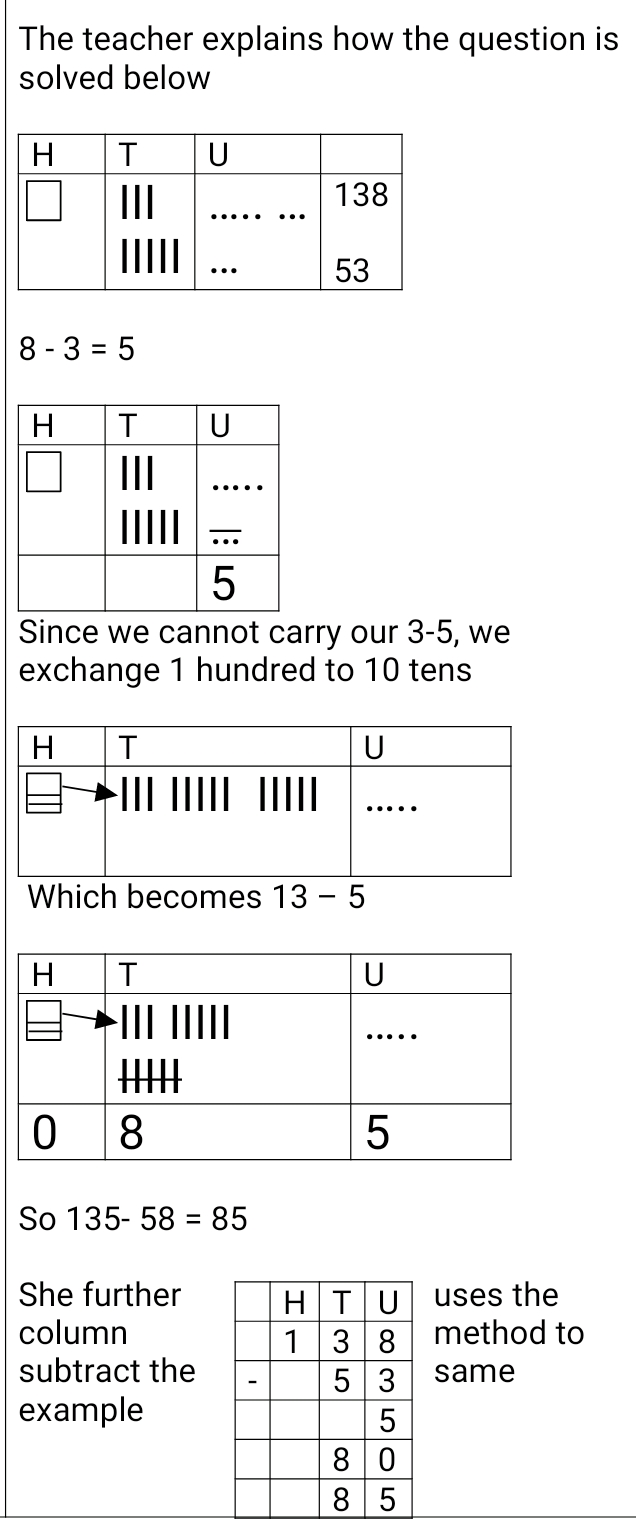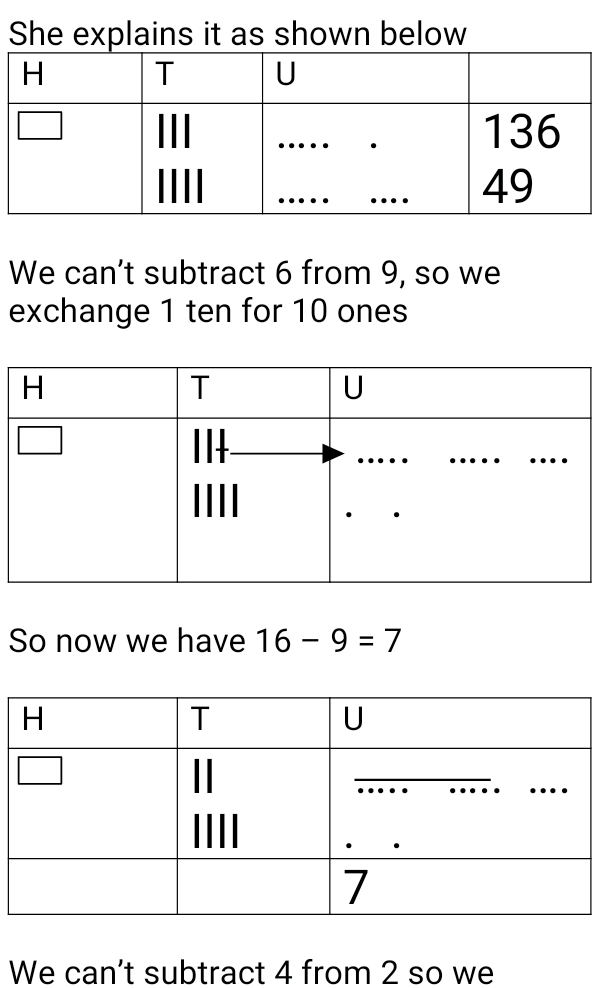# Lesson Notes By Weeks and Term - Primary 3

TERM: 1st Term

WEEK: 6

CLASS: Primary 3

AGE: 8 years

DURATION: 5 periods of 40 minutes each

DATE:

SUBJECT: Mathematics

SPECIFIC OBJECTIVES: At the end of the lesson, the pupils should be able to

1. Solve addition of 2-digit numbers using the column method

INSTRUCTIONAL TECHNIQUES: Explanation, question and answer, demonstration, practical, assessments

INSTRUCTIONAL MATERIALS: Base 10 kit, 100 number board, scrap paper, videos from source- https://www.youtube.com/watch?v=zyY0JLhA_9A

PERIOD 1: Addition using various strategies

 PRESENTATION TEACHER’S ACTIVITY PUPIL’S ACTIVITY STEP 1MENTAL MATHS The teacher begins the lesson with some mental calculationsCalculate1. 8 + _____ = 102. 37 + ___ = 403. 84 + _____= 904. 23 + _____ = 305. 51 + ______= 606. 75 +_____ = 807. 16 + _____ = 208. 42 + _____ = 509. 69 + _____ = 7010. 95 + ______ = Pupils respond and participate STEP 2CONCEPTDEVELOPMENT The teacher writes 98 + 34 = _____ on the board. Learners are encouraged to use any of the taught strategies to answer the questionsIn pairs, pupils are asked to work on the following sums using their preferred method56 + 75 = Pupils pay attention and participate STEP 3CLASS-WORK 1. Use column method and simplified pictorials to solve the followinga. 39 + 84 =b. 58 + 77 =c. 94 + 48 = Pupils attempt their class work STEP 4HOME-WORK 1. Use column method and simplified pictorials to solve the followinga. 86 + 65 =b. 67 + 96 = Pupils attempt their class work STEP 5SUMMARY The teacher summarizes reminding the pupils of the steps in carrying out addition using the simplified pictorial and the column method She marks their class works, makes corrections where necessary and commends them positively

 PRESENTATION TEACHER’S ACTIVITY PUPIL’S ACTIVITY STEP 1ORALASSESSMENTS The teacher asks questions on mental maths with 2-digit numbers treated so far Pupils respond and participate STEP 2DISCUSSION The teacher discusses all the methods used by some learners in the oral assessments(some of the questions are solved on the board by the learners) and addresses any misconceptions that may have risen Pupils pay attention and participate STEP 3WRITTEN ASSESSMENTS 1 Solve the following using simplified pictoriala. 65 + 52 =b. 54 + 85 =c. 39 + 94 =d. 86 + 98 =e. 58 + 59 = 2. Solve the following using the column methoda. 53 + 64=b. 81 + 73 =c. 85 + 89 =d. 57 + 86 =e. 74 + 48 = Pupils attempt their class work STEP 4SUMMARY The teacher marks the written assessments, corrects were necessary and commends the pupils

PERIOD 3: Subtraction using column method

PRESENTATION

TEACHER’S ACTIVITY

PUPIL’S ACTIVITY

STEP 1

MENTAL MATHS

The teacher begins the lesson with some mental calculations

Calculate

1. 13 - 5 =

2. 16 -  9 =

3. 15 - 9 =

4. 11 - 6=

5. 12 - 8=

6. 12 - 7 =

7. 16 - 7 =

8. 12 - 9 =

9. 12 - 6 =

10. 11 - 9 =

Pupils respond and participate

STEP 2

CONCEPT

DEVELOPMENT

The teacher writes 138 -53 = _____ on the board. The pupils are asked to solve the question using the simplified pictorial(hundreds, tens and ones should be crossed out using double lines and make sure arrows are drawn to signify borrowing)So 135- 58 = 85

She further uses the column method to subtract the same example

 H T U 1 3 8 - 5 3 5 8 0 8 5

O: 8-3 = 5

T: 130-50=80

Pupils pay attention and participate

STEP 3

CLASS-WORK

Solve the following using the column method

a. 159 – 64 =

b. 128 – 41 =

c. 136 – 82 =

d. 167 = 73 =

e. 119 – 36 =

Pupils attempt their class work

STEP 4

HOME-WORK

Solve the following using the column method

a. 155 – 92 =

b. 139 – 45 =

c. 147 - 74 =

The pupils writes it in their homework book

STEP 5

SUMMARY

The teacher reminds the pupils how simple subtraction problems were solved using pictorials and column method

She marks the class work, make corrections where necessary and commends the pupils positively

PERIOD 4: Subtraction using column method

 PRESENTATION TEACHER’S ACTIVITY PUPIL’S ACTIVITY STEP 1MENTAL MATHS The teacher begins the lesson with some mental calculationsCalculate1. 13 – 8 =2. 16 – 7 =3. 15 – 6 =4. 18 – 9 =5. 17 – 9 =6. 16 – 8 =7.  15 – 7 =8. 12 – 3 =9. 14 – 7 =10. 11 – 4 = Pupils respond and participate STEP 2CONCEPTDEVELOPMENT The teacher writes 136 – 49 on the board and asks the learners to solve the question using simplified pictorialsPupils pay attention and participate STEP 3CLASS-WORK Solve the following using column method and simplified pictorialsa. 114 - 28 =b. 144 – 67 =c. 123 - 98=d. 167 - 79 =e. 131 - 64= Pupils attempt their class work STEP 4HOME-WORK Solve the following using column method and simplified pictorialsa. 112 – 38 =b. 131 – 95c. 184 – 87 = The pupils writes it in their homework book STEP 5SUMMARY The teacher reminding the pupils how simple subtraction problems were solved using pictorials and column method She marks the class work, make corrections where necessary and commends the pupils positively

PERIOD 5: Weekly Test/consolidations

TEACHER’S ACTIVITY: The teacher revises all the concepts treated from period 1-4 and gives the pupils follow through exercises, quiz and tests . She marks the exercises, makes corrections and commends the pupils positively.

PUPIL’S ACTIVITY: The pupils work on the worksheets and exercises given by the teacher individually

ASSESSMENT

1 Solve the following using simplified pictorials:

a 81 + 76 = (157)

b 42 + 89 = (131)

c 175 – 82 = (93)

d 142 – 65 = (77)

2 Solve the following using the column method:

a 56 + 75 = (131)

b 99 + 99 = (198)

c 113 – 48 = (65)

d 162 – 88 = (74)